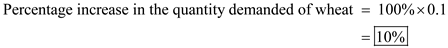## Quiz 19 : Antitrust Policy and RegulationLooking for Economics Homework Help?# Quiz 19 : Antitrust Policy and Regulation

a) We are given that the price elasticity of corn is 0.1 and the price elasticity of wheat is 0.15. So, percentage change in quantity of corn is calculated using the formula of price elasticity given below: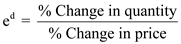. We are given the price elasticity, or e d , and the % change in price, and we need to find out the % change in quantity. Thus, for corn, if prices fell by 25% and the price elasticity is.1, we have:Thus, the percentage increase for the demand of corn iS2.5%. If the price of corn were to fall by 50% instead of 25%, then, % change in quantity of corn will be calculated as follows:(b) We are given that the price elasticity of wheat is 0.15, and we want the % change in quantity for wheat to be 5%. Therefore, it will be calculated by using the following formula:Thus, prices must change by 33.33% for consumers to increase their purchases of wheat by 5 percent. (c) If the price of corn increases by 10 percent from the tax, then % change in quantity will be calculated as follows:Thus, we see that with a 10 percent change in price, there will be a 1 percent change in quantity demanded. If originally, the quantity demanded iS10 billion bushels per year, then the tax will cause a 1 percent decrease in the quantity demanded. One percent of 10 billion iS100 million, so, the tax will end up causing quantity demanded of corn to fall by 100 million bushels.

(a)    A decreasing rate; because marginal utility is declining. (b)   Disagree.  The marginal utility of a unit beyond the first may be sufficiently great (relative to product price) to make it a worthwhile purchase.  Consumers are interested in maximizing total utility, not marginal utility. (c) Agree.  This product's price could be so high relative to the first unit's marginal utility that the consumer would buy none of it.

(a) Percentage increase in the income = 2%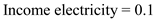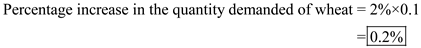[It should be recalled that there is a direct relationship between income and quantity demanded. An increase in any variable implies +ve sign and a decrease imply -ve sign.]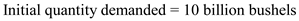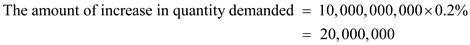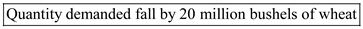________________________________________________________________________ (b) Percentage increase in the income = 100% Income electricity = 0.1